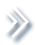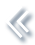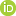####ArchivesReview Articles

## Model averaging for generalized linear models in fragmentary data prediction

Chaoxia Yuan ,

KLATASDS – MOE, School of Statistics, East China Normal University, Shanghai, People's Republic of Chinachaoxiayuan@163.com

Yang Wu ,

KLATASDS – MOE, School of Statistics, East China Normal University, Shanghai, People's Republic of ChinaFang Fang

KLATASDS – MOE, School of Statistics, East China Normal University, Shanghai, People's Republic of ChinaPages | Received 01 Feb. 2022, Accepted 18 Jul. 2022, Published online: 30 Jul. 2022,
• Abstract
• References
• Citations

Fragmentary data is becoming more and more popular in many areas which brings big challenges to researchers and data analysts. Most existing methods dealing with fragmentary data consider a continuous response while in many applications the response variable is discrete. In this paper, we propose a model averaging method for generalized linear models in fragmentary data prediction. The candidate models are fitted based on different combinations of covariate availability and sample size. The optimal weight is selected by minimizing the Kullback–Leibler loss in the completed cases and its asymptotic optimality is established. Empirical evidences from a simulation study and a real data analysis about Alzheimer disease are presented.

• Akaike, H. (1970). Statistical predictor identification. Annals of the Institute of Statistical Mathematics22(1), 203–217. https://doi.org/10.1007/BF02506337
• Ando, T., & Li, K.-C. (2014). A model averaging approach for high dimensional regression. Journal of American Statistical Association109(505), 254–265. https://doi.org/10.1080/01621459.2013.838168
• Ando, T., & Li, K.-C. (2017). A weight-relaxed model averaging approach for high-dimensional generalized linear models. The Annals of Statistics45(6), 2654–2679. https://doi.org/10.1214/17-AOS1538
• Buckland, S. T., Burnham, K. P., & Augustin, N. H. (1997). Model selection: An integral part of inference. Biometrics53(2), 603–618. https://doi.org/10.2307/2533961
• Chen, J., Li, D., Linton, O., & Lu, Z. (2018). Semiparametric ultra-high dimensional model averaging of nonlinear dynamic time series. Journal of the American Statistical Association113(522), 919–932. https://doi.org/10.1080/01621459.2017.1302339
• Dardanoni, V., Luca, G. D., Modica, S., & Peracchi, F. (2015). Model averaging estimation of generalized linear models with imputed covariates. Journal of Econometrics184(2), 452–463. https://doi.org/10.1016/j.jeconom.2014.06.002
• Dardanoni, V., Modica, S., & Peracchi, F. (2011). Regression with imputed covariates: A generalized missing indicator approach. Journal of Econometrics162(2), 362–368. https://doi.org/10.1016/j.jeconom.2011.02.005
• Ding, X., Xie, J., & Yan, X. (2021). Model averaging for multiple quantile regression with covariates missing at random. Journal of Statistical Computation and Simulation91(11), 2249–2275. https://doi.org/10.1080/00949655.2021.1890733
• Fan, J., & Li, R. (2001). Variable selection via nonconcave penalized likelihood and its oracle properties. Journal of American Statistical Association96(456), 1348–1360. https://doi.org/10.1198/016214501753382273
• Fan, J., & Lv, J. (2008). Sure independence screening for ultrahigh dimensional feature space (with discussions). Journal of the Royal Statistical Society: Series B (Statistical Methodology)70(5), 849–911. https://doi.org/10.1111/rssb.2008.70.issue-5
• Fang, F., Li, J., & Xia, X. (2022). Semiparametric model averaging prediction for dichotomous response. Journal of Econometrics229(2), 219–245. https://doi.org/10.1016/j.jeconom.2020.09.008
• Fang, F., Wei, L., Tong, J., & Shao, J. (2019). Model averaging for prediction with fragmentary data. Journal of Business & Economic Statistics37(3), 517–527. https://doi.org/10.1080/07350015.2017.1383263
• Hansen, B. E. (2007). Least squares model averaging. Econometrica75(4), 1175–1189. https://doi.org/10.1111/ecta.2007.75.issue-4
• Hansen, B. E., & Racine, J. S. (2012). Jackknife model averaging. Journal of Econometrics167(1), 38–46. https://doi.org/10.1016/j.jeconom.2011.06.019
• Hjort, N. L., & Claeskens, G. (2003). Frequentist model average estimators. Journal of American Statistical Association98(464), 879–899. https://doi.org/10.1198/016214503000000828
• Hoeting, J., Madigan, D., Raftery, A., & Volinsky, C. (1999). Bayesian model averaging: A tutorial. Statistical Science14(4), 382–401. https://doi.org/10.1214/ss/1009212519
• Kim, J. K., & Shao, J. (2013). Statistical methods for handling incomplete data. Chapman & Hall/CRC.
• Leung, G., & Barron, A. R. (2006). Information theory and mixing least-squares regressions. IEEE Transactions on Information Theory52(8), 3396–3410. https://doi.org/10.1109/TIT.2006.878172
• Li, C., Li, Q., Racine, J. S., & Zhang, D. (2018). Optimal model averaging of varying coefficient models. Statistica Sinica28(2), 2795–2809. https://doi.org/10.5705/ss.202017.0034
• Li, D., Linton, O., & Lu, Z. (2015). A flexible semiparametric forecasting model for time series. Journal of Econometrics187(1), 345–357. https://doi.org/10.1016/j.jeconom.2015.02.025
• Liao, J., Zong, X., Zhang, X., & Zou, G. (2019). Model averaging based on leave-subject-out cross-validation for vector autoregressions. Journal of Econometrics209(1), 35–60. https://doi.org/10.1016/j.jeconom.2018.10.007
• Lin, H., Liu, W., & Lan, W. (2021). Regression analysis with individual-specific patterns of missing covariates. Journal of Business & Economic Statistics39(1), 179–188. https://doi.org/10.1080/07350015.2019.1635486
• Little, R. J. A., & Rubin, D. B. (2002). Statistical analysis with missing data. 2nd ed. Wiley.
• Liu, Q., & Okui, R. (2013). Heteroskedasticity-robust CpCp model averaging. The Econometrics Journal16(3),463–472. https://doi.org/10.1111/ectj.12009
• Liu, Q., & Zheng, M. (2020). Model averaging for generalized linear model with covariates that are missing completely at random. The Journal of Quantitative Economics11(4), 25–40. https://doi.org/10.16699/b.cnki.jqe.2020.04.003
• Longford, N. T. (2005). Editorial: Model selection and efficiency is ‘Which model…?’ the right question? Journal of the Royal Statistical Society: Series A (Statistics in Society)168(3), 469–472. https://doi.org/10.1111/rssa.2005.168.issue-3
• Lu, X., & Su, L. (2015). Jackknife model averaging for quantile regressions. Journal of Econometrics188(1), 40–58. https://doi.org/10.1016/j.jeconom.2014.11.005
• Mallows, C. (1973). Some comments on CpCp. Technometrics15(4), 661–675. https://doi.org/10.2307/1267380
• Meier, L., Geer, S. V. D., & Peter, B. (2008). The group lasso for logistic regression. Journal of the Royal Statistical Society: Series B (Statistical Methodology)70(1), 53–71. https://doi.org/10.1111/j.1467-9868.2007.00627.x
• Schomaker, M., Wan, A. T. K., & Heumann, C. (2010). Frequentist model averaging with missing observations. Computational Statistics and Data Analysis54(12), 3336–3347. https://doi.org/10.1016/j.csda.2009.07.023
• Schwarz, G. (1978). Estimating the dimension of a model. The Annals of Statistics6(2), 461–464. https://doi.org/10.1214/aos/1176344136
• Tibshirani, R. (1996). Regression shrinkage and selection via the lasso. Journal of the Royal Statistical Society: Series B (Methodological)58(1), 267–288. https://doi.org/10.1111/j.2517-6161.1996.tb02080.x
• Wan, A. T. K., Zhang, X., & Zou, G. (2010). Least squares model averaging by Mallows criterion. Journal of Econometrics156(2), 277–283. https://doi.org/10.1016/j.jeconom.2009.10.030
• White, H. (1982). Maximum likelihood estimation of misspecified models. Econometrica50(1), 1–25. https://doi.org/10.2307/1912526
• Xue, F., & Qu, A. (2021). Integrating multi-source block-wise missing data in model selection. Journal of American Statistical Association116(536), 1914–1927. https://doi.org/10.1080/01621459.2020.1751176
• Yang, Y. (2001). Adaptive regression by mixing. Journal of American Statistical Association96(454), 574–588. https://doi.org/10.1198/016214501753168262
• Yang, Y. (2003). Regression with multiple candidate models: Selecting or mixing? Statistica Sinica13, 783–809.
• Zhang, X. (2013). Model averaging with covariates that are missing completely at random. Economics Letters121(3), 360–363. https://doi.org/10.1016/j.econlet.2013.09.008
• Zhang, X., Yu, D., Zou, G., & Liang, H. (2016). Optimal model averaging estimation for generalized linear models and generalized linear mixed-effects models. Journal of the American Statistical Association111(516), 1775–1790. https://doi.org/10.1080/01621459.2015.1115762
• Zhang, X., Zou, G., & Liang, H. (2014). Model averaging and weight choice in linear mixed effects models. Biometrika101(1), 205–218. https://doi.org/10.1093/biomet/ast052
• Zhang, X., Zou, G., Liang, H., & Carroll, R. J. (2020). Parsimonious model averaging with a diverging number of parameters. Journal of the American Statistical Association115(530), 972–984. https://doi.org/10.1080/01621459.2019.1604363
• Zhang, Y., Tang, N., & Qu, A. (2020). Imputed factor regression for high-dimensional block-wise missing data. Statistica Sinica30(2), 631–651. https://doi.org/10.5705/ss.202018.0008
• Zheng, H., Tsui, K-W, Kang, X., & Deng, X. (2017). Cholesky-based model averaging for covariance matrix estimation. Statistical Theory and Related Fields1(1), 48–58. https://doi.org/10.1080/24754269.2017.1336831
• Zhu, R., Wan, A. T. K., Zhang, X., & Zou, G. (2019). A Mallow-type model averaging estimator for the varying-coefficient partially linear model. Journal of the American Statistical Association114(526), 882–892. https://doi.org/10.1080/01621459.2018.1456936

To cite this article: Chaoxia Yuan, Yang Wu & Fang Fang (2022): Model averaging for generalized linear models in fragmentary data prediction, Statistical Theory and Related Fields, DOI: 10.1080/24754269.2022.2105486